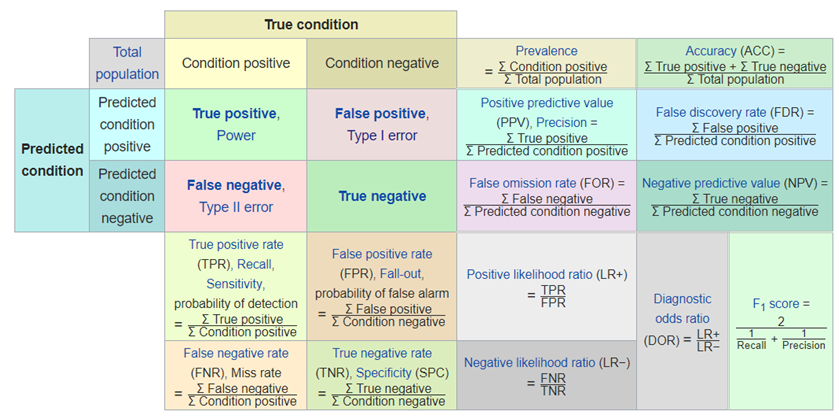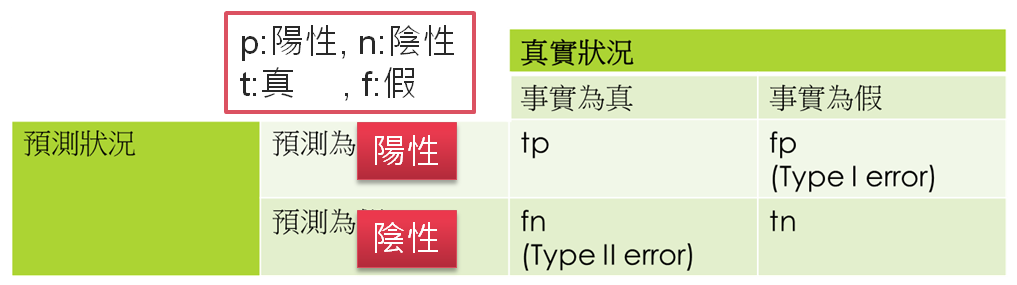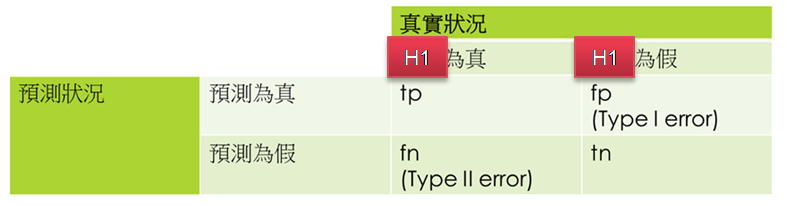#2

## 前言

1. 混淆矩陣(Confusion Matrix)。
2. 準確率(Accuracy)、精確率(Precision)、召回率(Recall)、F1 Score、真陽率(True Positive Rate)、假陽率(False Positive Rate)...。
3. ROC/AUC 曲線## 混淆矩陣(Confusion Matrix)1. tp：預測為陽性(p)，預測正確(t)。
2. tn：預測為陰性(n)，預測正確(t)。
3. fp：預測為陽性(p)，預測錯誤(f)。
4. fn：預測為陰性(n)，預測錯誤(f)。## 混淆矩陣計算範例

``````from sklearn.metrics import confusion_matrix

actual = [1, 1, 0, 1, 0, 0, 1, 0, 0, 0]
predicted = [1, 0, 0, 1, 0, 0, 1, 1, 1, 0]
tn, fp, fn, tp = confusion_matrix(actual, predicted).ravel()
print(tn, fp, fn, tp)
``````

## 準確率、精確率(Precision)、召回率(Recall)、F1 Score

F1 = 2 / ( (1/ Precision) + (1/ Recall) )，即精確率與召回率的調和平均數。

1. 未故障： 2/3
2. 故障時：0/0，無法計算。
應該就可以發現設備有問題了。